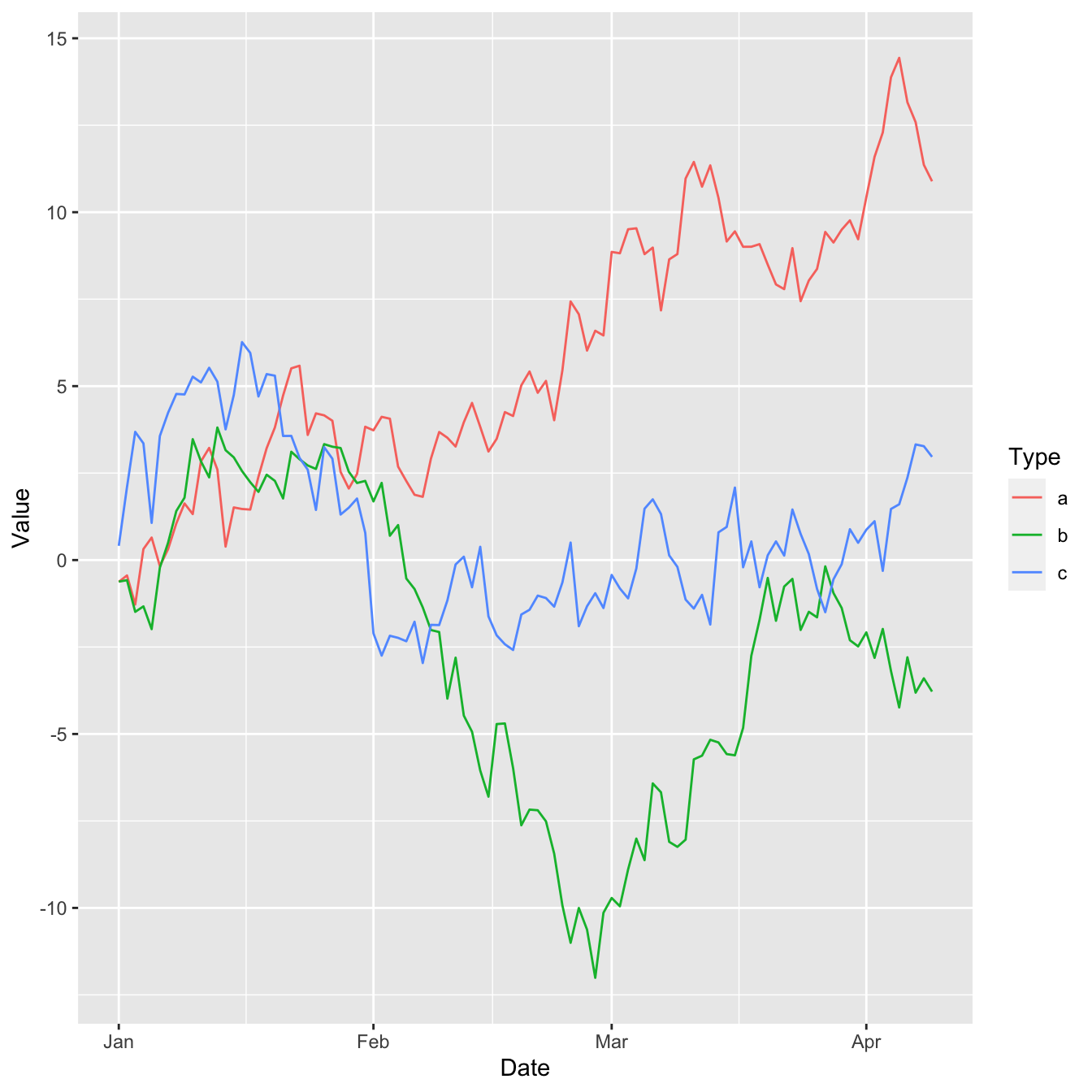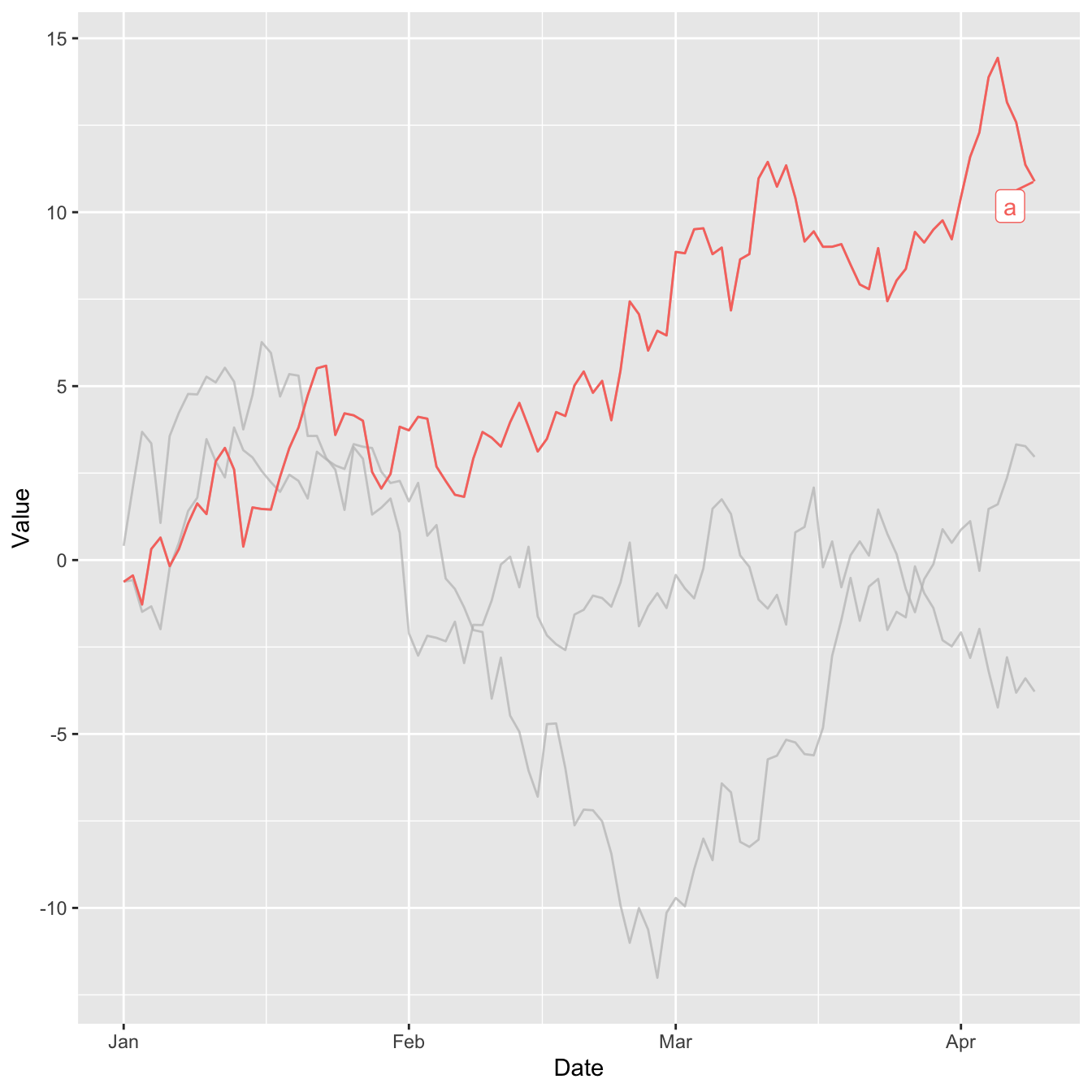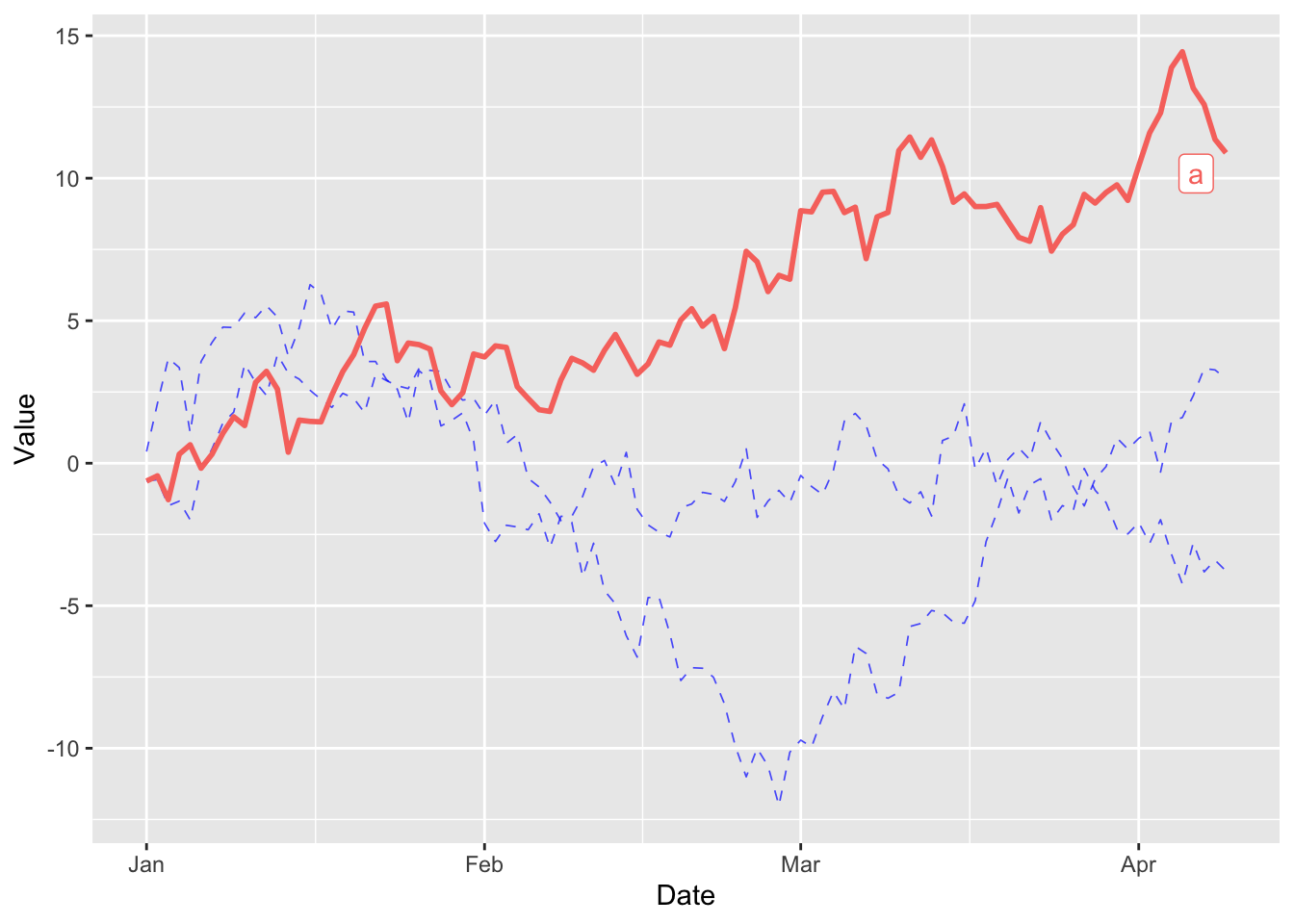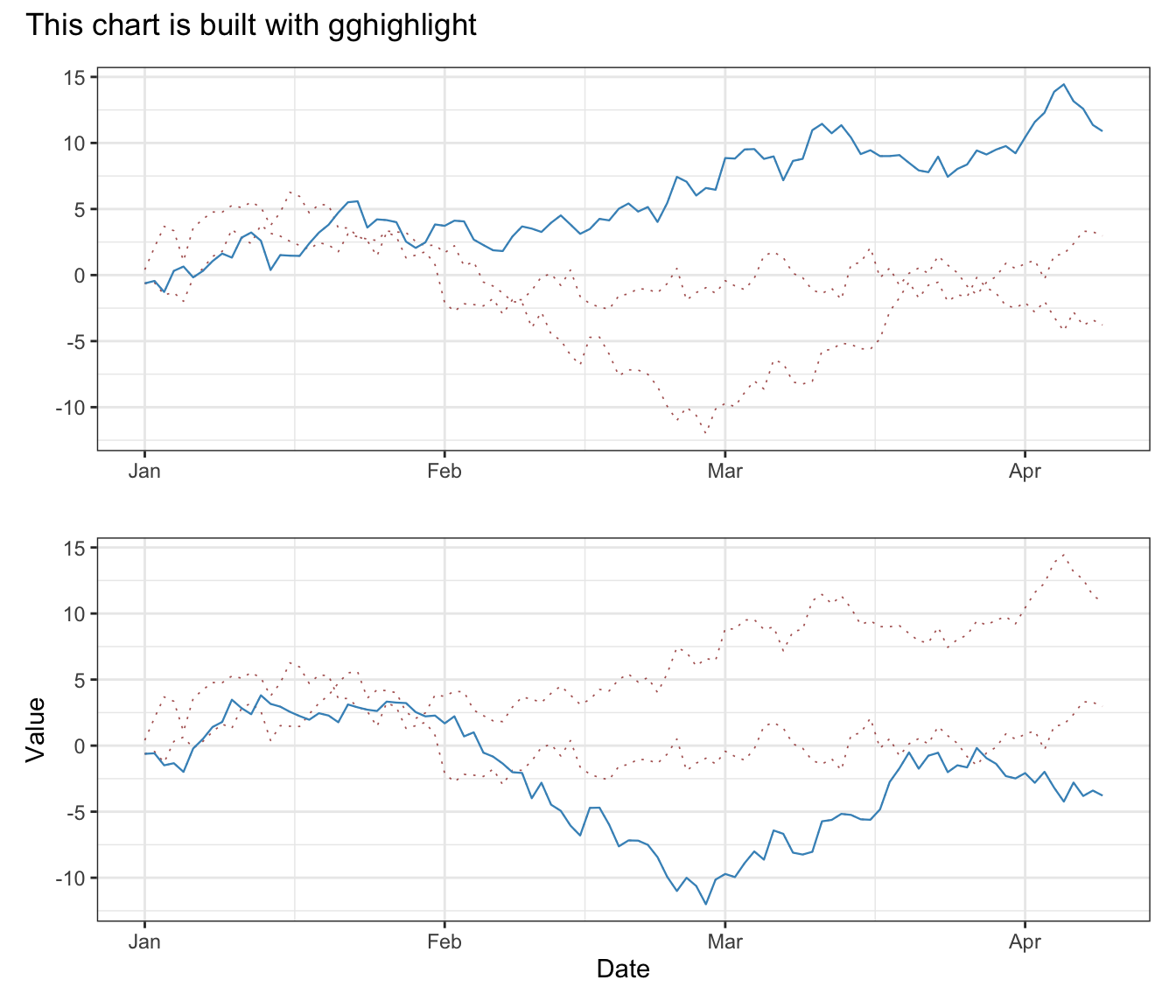# Highlight specific elements in a line chart

This post explains how to highlight elements in a line chart with ggplot2 and gghighlight. Highlighting elements on a chart is a very powerful dataviz technique. It leads the reader to the important message you are trying to convey, making the figure more insightful.

# Dataset

The dataset has three columns:

• `Date`: This column contains dates starting from January 1, 2020, and continuing for 100 days.

• `Value`: This column holds numbers. These numbers are created by adding random amounts to each other in a cumulative way. It’s like keeping a running total of random values.

• `Type`: This column categorizes each row with one of three labels: `a`, `b`, or `c`.

``````# dataset with 3 lines named "a", "b" and "c"
set.seed(1)
period = 100
df = data.frame(Date = seq(as.Date("2020-01-01"),
by = "day",
length.out = period),
Value = c(cumsum(rnorm(period)),
cumsum(rnorm(period)),
cumsum(rnorm(period))),
Type = c(rep("a", period),
rep("b", period),
rep("c", period)))``````

# Package

For this post, we need the ggplot2 and gghighlight packages.

``````# Install the packages if not already done
# install.packages("ggplot2")
# install.packages("gghighlight")

library(ggplot2)
library(gghighlight)``````

# Default line chart in ggplot2

Here’s what the default line chart output looks like with `ggplot2`:# Highlight specific lines

With the `gghighlight()` function and some criterion, we can indicate which line will be displayed.

In this case, it’s all lines where the maximum `Value` is greater than 10.

``````ggplot(df) +
geom_line(aes(Date, Value, colour = Type)) +
gghighlight(max(Value) > 10)``````# Properties of faded out lines

The `gghighlight()` function has an argument named unhighlighted_params that allows us to define what are the properties to use for the lines that does not meet our criterion.

In this case, we reduce the line width, change the color to blue and lower the opacity (`alpha`). We also change the line to `dashed`.

``````ggplot(df) +
geom_line(aes(Date, Value, colour = Type), linewidth=1) +
gghighlight(max(Value) > 10,
unhighlighted_params = list(linewidth = 0.3,
colour = alpha("blue", 0.7),
linetype = "dashed"))``````# A more complex example

The `gghighlight` package is also very useful when combined with other packages such as hrbrthemes and patchwork.

In this example we use the power of `gghighlight` with the simplicity of `patchwork` for stacking graphs and `hrbrthemes'` beautiful themes.

``````library(hrbrthemes)
library(patchwork)

plot1 = ggplot(df) +
geom_line(aes(Date, Value, colour = Type), linewidth=0.4, color='#4393C3') +
gghighlight(max(Value) > 10,
unhighlighted_params = list(linewidth = 0.3,
colour = alpha("darkred", 0.7),
linetype = "dotted"),
use_direct_label = FALSE) +
theme_bw() + xlab("") + ylab("")

plot2 = ggplot(df) +
geom_line(aes(Date, Value, colour = Type), linewidth=0.4, color='#4393C3') +
gghighlight(min(Value) < -10,
unhighlighted_params = list(linewidth = 0.3,
colour = alpha("darkred", 0.7),
linetype = "dotted"),
use_direct_label = FALSE) +
theme_bw()

plot1 / plot2 + plot_annotation(title = 'This chart is built with gghighlight')``````# Conclusion

In this post, we look at how to use the gghighlight package to highlight specific elements of a multi-part line chart. To find out more about how to customize a line chart, see the dedicated section.

Related chart types

## Contact

This document is a work by Yan Holtz. Any feedback is highly encouraged. You can fill an issue on Github, drop me a message on Twitter, or send an email pasting yan.holtz.data with gmail.com.